Maths (41) reasoning (32) Home (16) integration (6)

## Ten Missing number questions with solutions for ssc cgl exam

Ten Missing number questions with solutions for ssc cgl exam are discussed in this post . These questions of reasoning in latest reasoning questions with answers are very very important for upcoming competitive exams  like Bank PO , SSC CGL etc . So let us start solving and understanding these Maths logical reasoning questions with answers.

## Problem # 1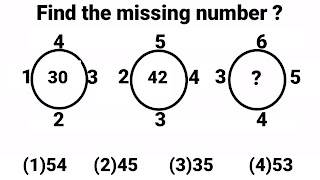Exam Cracker
There are three circles in this reasoning problem and every circle have five numbers associated to it. Out of these five numbers four are placed around the circle and fifth is in the centre of the circle. In the 3rd circle the value of question mark will be calculated using all the four associated numbers using the same formula which have been used in the 1st and 2nd circle to calculate the value of middle number.
Formula :- 3 times the sum of four numbers around the circle
3 (4 + 3 + 2  + 1 ) = 3 × 10 = 30  (  middle number in 1st figure  )
3 ( 5 + 4  + 3 + 2  ) = 3 × 14 = 42  ( middle number in 2nd figure  )
3 ( 6 + 5 + 4 + 3 ) = 3 × 18 = 54  (middle number in 3rd figure)
Option (1)54 is correct option

## Problem # 2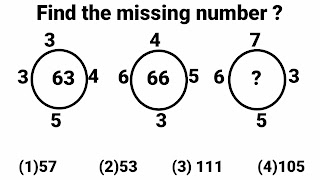Exam Cracker
There are three circles in this reasoning problem and every circle have five numbers associated to it. Out of these five numbers four are placed around the circle and fifth is in the centre of the circle. In the 3rd circle the value of question mark will be calculated using all the four associated numbers using the same formula which have been used in the 1st and 2nd circle to calculate the value of middle number.
Formula :- Left number + ( Product of remaining three numbers ) = Middle number
3 + ( 3 × 4 × 5 ) = 3 + 60 =  63 ( middle number in 1st figure  )
6 + ( 4 × 5 × 3) = 6 + 60 = 66 ( middle number in 1st figure  )
6 + ( 7 × 3 × 5 ) = 6 + 105 =  111 ( middle number in 1st figure  )
Option (1)71 is correct option

## Problem # 3Exam Cracker
There are three circles in this reasoning problem and every circle have five numbers associated to it. Out of these five numbers four are placed around the circle and fifth is in the centre of the circle. In the 3rd circle the value of question mark will be calculated using all the four associated numbers using the same formula which have been used in the 1st and 2nd circle to calculate the value of middle number.

Formula :- Sum of squares of four numbers around the circle is  = Middle number

1² + 4² + 3² + 2² = 1 + 16 + 9 + 4 = 30 ( middle number in 1st figure )
2² + 5² + 4² + 3² = 4 + 25 + 16 + 9 = 54 ( middle number in 2nd figure )
3² + 6² + 5² + 4² = 9 + 36 + 25 + 16 = 86 ( middle number in 3rd figure )
Option (1)86 is correct option

## Problem # 4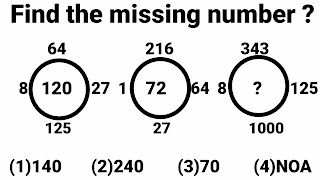Exam Cracker
There are three circles in this reasoning problem and every circle have five numbers associated to it. Out of these five numbers four are placed around the circle and fifth is in the centre of the circle. In the 3rd circle the value of question mark will be calculated using all the four associated numbers using the same formula which have been used in the 1st and 2nd circle to calculate the value of middle number.
Formula :- Product of cube root of four numbers around the circle  = Middle number
∛8 ×∛64 ×∛27 ×∛125 = 2 × 4 × 3 × 5 = 120 ( Middle number in 1st circle)
∛1 ×∛216 ×∛64 ×∛27 = 1 × 6 × 4 × 3 = 72 ( Middle number in 2nd circle)
∛8 ×∛343 ×∛125 ×∛1000 = 2 × 7 × 5 × 10 = 700 ( Middle number in 3rd circle) = The value of question mark, But 700 is not in any of three options. Therefore
Option (4)NOA is correct option

## Problem # 5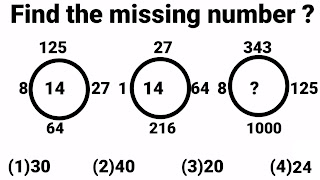Exam Cracker
There are three circles in this reasoning problem and every circle have five numbers associated to it. Out of these five numbers four are placed around the circle and fifth is in the centre of the circle. In the 3rd circle the value of question mark will be calculated using all the four associated numbers using the same formula which have been used in the 1st and 2nd circle to calculate the value of middle number.
Formula :- Sum of cube root of four numbers around the circle = Middle number
∛8 + ∛64 + ∛27 + ∛125 = 2 + 4 + 3 + 5 = 14 ( Middle number in 1st circle)
∛1 + ∛216 + ∛64 + ∛27 = 1 + 6 + 4 + 3 = 14 ( Middle number in 2nd circle)
∛8 + ∛343  + ∛125 + ∛1000 = 2 + 7 + 5 + 10 = 24 ( Middle number in 3rd circle) = The value of question mark. Therefore
Option (4)24 is correct option

## Problem # 6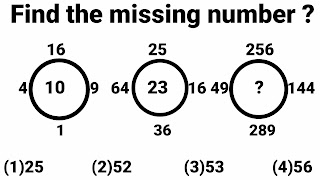Exam Cracker
There are three circles in this reasoning problem and every circle have five numbers associated to it. Out of these five numbers four are placed around the circle and fifth is in the centre of the circle. In the 3rd circle the value of question mark will be calculated using all the four associated numbers using the same formula which have been used in the 1st and 2nd circle to calculate the value of middle number.
Formula :- Sum of square root of four numbers around the circle   = Middle number
√4+ √16 + √9 + √1 = 2 + 4 + 3 + 1 = 10 ( Middle number in 1st circle)
√64+ √25 + √16 + √36 = 8 + 5 + 4 + 6 = 23 ( Middle number in 2nd circle)
√49+ √256 + √144 + √289 = 7 + 16 + 12 + 17 = 52 ( Middle number in 3rd circle)
Option (2)52 is correct option

## Problem # 7Exam Cracker
There are three circles in this reasoning problem and every circle have five numbers associated to it. Out of these five numbers four are placed around the circle and fifth is in the centre of the circle. In the 3rd circle the value of question mark will be calculated using all the four associated numbers using the same formula which have been used in the 1st and 2nd circle to calculate the value of middle number.
Formula :- Product of square root of four numbers around the circle  = Middle number
√4× √16 × √9 × √1 = 2 × 4 × 3 × 1 = 24 ( Middle number in 1st circle)
√64+ √25 + √16 + √36 = 8 × 5 × 4 × 6 = 960 ( Middle number in 2nd circle)
√9 + √100+ √16 + √121 = 3 × 10 × 4 × 11 = 1320 ( Middle number in 3rd circle)
Option (2)1320 is correct option

## Problem # 8Exam Cracker
There are three circles in this reasoning problem and every circle have five numbers associated to it. Out of these five numbers four are placed around the circle and fifth is in the centre of the circle. In the 3rd circle the value of question mark will be calculated using all the four associated numbers using the same formula which have been used in the 1st and 2nd circle to calculate the value of middle number.
Formula :- Sum of square root of cube root of four numbers around the circle   = Middle number
(∛8)² + (∛125)² + (∛27)² + (∛64)² = 2² + 5² + 3² + 4² =  4 + 25 + 9 + 16 = 54 ( Middle number in 1st circle)
(∛1) ² + (∛27)² + (∛64)² + (∛216)²= 1² + 3² + 4² + 6² = 1 + 9 + 16 + 36 = 62  ( Middle number in 2nd circle)
(∛8) ² + (∛343)² + (∛125)² + (∛100)²= 2² + 7² + 5² + 10² = 4 + 49 + 25 + 100 = 178 ( Middle number in 3rd circle)  = The value of question mark. Therefore
Option (4)178 is correct option

StartExam Cracker
There are three circles in this reasoning problem and every circle have five numbers associated to it. Out of these five numbers four are placed around the circle and fifth is in the centre of the circle. In the 3rd circle the value of question mark will be calculated using all the four associated numbers using the same formula which have been used in the 1st and 2nd circle to calculate the value of middle number.
Formula :- Highest common factor of four numbers around the circle  = Middle number
HCF of  9, 12, 6 and 18 = 3
HCF of 30, 50, 60 and 20 = 10
HCF of  3, 5, 6 and 2 = 1 = The value of question mark.
Option (4)NOA is correct option

## Problem # 10Exam Cracker
There are three circles in this reasoning problem and every circle have five numbers associated to it. Out of these five numbers four are placed around the circle and fifth is in the centre of the circle. In the 3rd circle the value of question mark will be calculated using all the four associated numbers using the same formula which have been used in the 1st and 2nd circle to calculate the value of middle number.
Formula :- Least common multiple of four numbers around the circle  = Middle number
LCM of  3, 2, 6 and 8 = 24
LCM of 3, 5, 6 and 10 = 30
LCM of  3, 9, 6 and 2 = 18 = The value of question mark.
Option (1)18 is correct option

Comment your valuable suggestion regarding the post "most important Reasoning questions with answers" which includes reasoning for competitive exams, circle problems, box problems, circle problems and triangles problems  for competitive exams like SSC CGL ,SSC CHSL ,CPO ,Bank exams and RRB NTPC etc which were explained in this post.

Share: